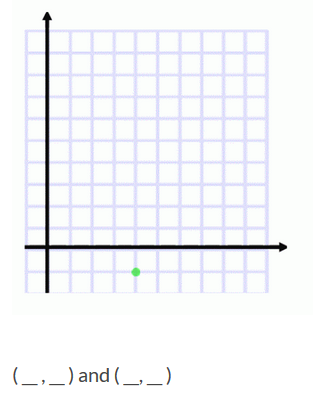# Equidistant Points 2

Directions: Using the digits 1 to 9 at most one time each, fill in the boxes to create two points that are equidistant from (4,-1).### Hint

Which methods are available to determine answers to this problem? What shape is defined by all of the points that are equidistant from (4, -1)?

### Answer

There are many correct answers to this problem including: (2, 4) and (9, 1) as well as (2, 3), and (8, 1)

Source: Bryan Anderson

## Pythagorean Inequality

Directions: Using the digits 1 through 6 at most one time each, fill in the …

### 2 comments

1.We worked this problem out and only found 4 possible answers. Do you think there are more than that?

Our other answers were (1,4), (9,2) and (1,3), (8,2).

Thanks!

•there are many more, if you think in terms of slope, any that create perpendicular lines will work; all the points to the left or right two and up one will be the same as going left or right one, then up two. etc